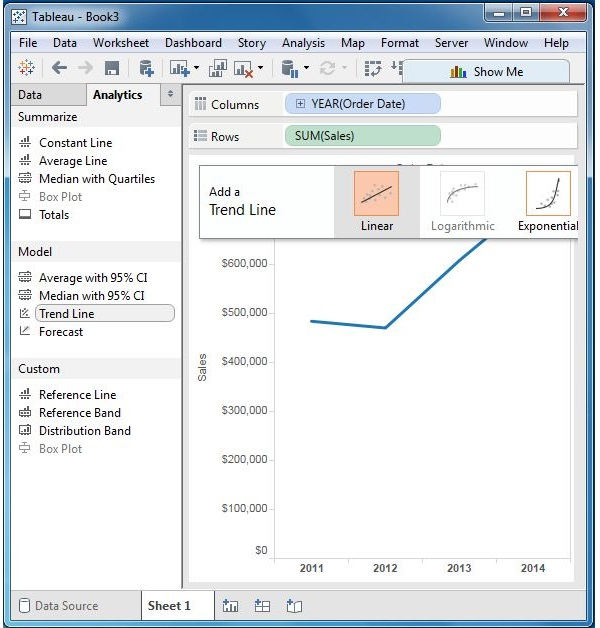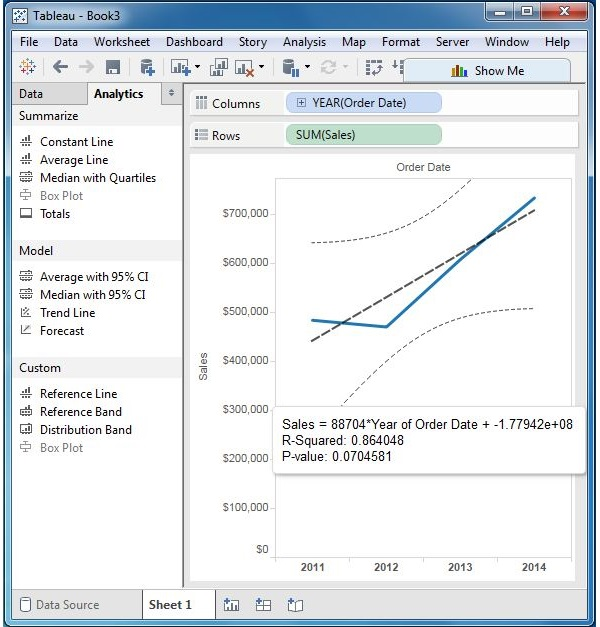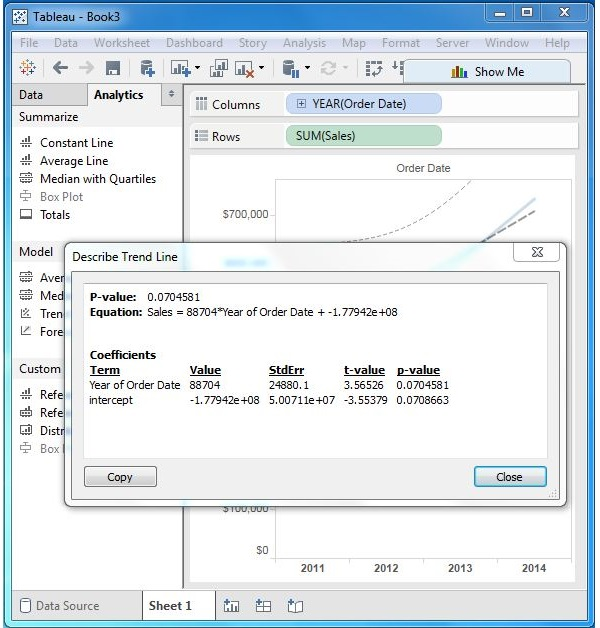# Tableau - Trend Lines

Trend lines are used to predict the continuation of a certain trend of a variable. It also helps to identify the correlation between two variables by observing the trend in both of them simultaneously. There are many mathematical models for establishing trend lines. Tableau provides four options. They are Linear, Logarithmic, Exponential, and Polynomial. In this chapter, only the linear model is discussed.

Tableau takes a time dimension and a measure field to create a Trend Line.

## Creating a Trend Line

Using the Sample-superstore, find the trend for the value of the measure sales for next year. To achieve this objective, following are the steps.

Step 1 − Drag the dimension Order date to the Column shelf and the measure Sales to the Rows shelf. Choose the chart type as Line chart. In the Analysis menu, go to model → Trend Line. Clicking on Trend Line pops up an option showing different types of trend lines that can be added. Choose the linear model as shown in the following screenshot.Step 2 − On completion of the above step, you will get various trend lines. It also shows the mathematical expression for the correlation between the fields, the P-Value and the R-Squared value.### Describe the Trend Line

Right-click on the chart and select the option Describe Trend Line to get a detailed description of the Trend Line chart. It shows the coefficients, intercept value, and the equation. These details can also be copied to the clipboard and used in further analysis.